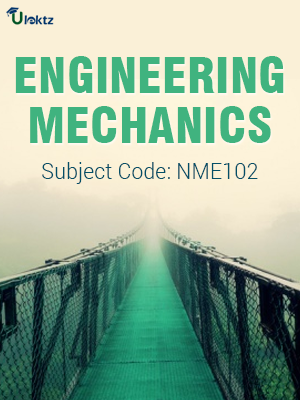uLektz apps

•My WalletMy Order
•My Profile
•My Connections
•My Books
•My Videos
•My Tests
•My Calender
•My Messages
•My Shopping Cart
•My Orders
•Account Settings
•Help

uLektz apps

Book DetailsEngineering Mechanics

Course Code:NME102

Author:uLektz

Regulation:2010

Categories:Mechanical

Format :ePUB3 (DRM Protected)

Type :eBook

FREE

Description :Engineering Mechanics of NME102 covers the latest syllabus prescribed by Dr. A.P.J. Abdul Kalam Technical University, Uttar Pradesh for regulation 2010. Author: uLektz, Published by uLektz Learning Solutions Private Limited.

Note : No printed book. Only ebook. Access eBook using uLektz apps for Android, iOS and Windows Desktop PC.

Topics
Unit 1 Force Systems, Friction

1.1 Basic concepts: Definitions, Basic assumptions, Scalar & Vector quantities, Free, Forced and fixed vectors.

1.2 Force System: Force, Classification & Representation, Force as a Vector,

1.3 Composition of forces, Parallelogram Law, Resolution, Principle of Transmissibility of forces

1.4 Moment of a force, Vector representation, Moment for coplanar force system, Varignon’s theorem

1.5 Couple, Vector representation, Resolution of a force into a force and a couple.

1.6 Force Systems: Coplanar Concurrent Force system and Coplanar Non Concurrent force systems, Resultant of coplanar force system.

1.7 Equilibrium of coplanar force system, Free body diagrams, Determination of reactions, Equilibrium of a body under three forces, Lami’s theorem.

1.8 Introduction, Wet and Dry friction, Theory of Dry friction, Angle of friction,

1.9 Angle of Repose, Cone of friction, Coulomb’s laws of friction.

Unit 2 Basic Structural Analysis

2.1 Plane Truss, Difference between truss and frame, Perfect and imperfect truss, Assumptions and Analysis of Plane Truss ,

2.2 Method of joints,

2.3 Method of section, Zero force members.

2.4 Beams, Types of beams, Statically Determinate Beams,

2.5 Shear force and bending moment in beams, Shear force and bending moment diagrams, Relationships between load, shear and bending moment

Unit 3 Centroid and Moment of Inertia

3.1 Center of Gravity, Center of Mass and Centroid of curves, areas, volumes,

3.2 Determination of centroid by integration, Centroid of composite bodies.

3.3 Definition of Moment of inertia of area, Perpendicular axis theorem and Polar moment of Inertia,

3.4 Parallel axis theorem, Moment of inertia of simple areas by integration, Moment of Inertia of Composite Areas.

3.5 Moment of Inertia of masses, Parallel axis theorem for mass moment of inertia,

3.6 Mass moment of inertia of simple bodies by integration, Mass moment of inertia of composite bodies.

Unit 4 Kinematics of Rigid Body, Kinetics of Rigid Body

4.1 Introduction, Absolute motion, Plane rectilinear motion of rigid body

4.2 Plane curvilinear Motion of rigid body, x-y and n-t components, Rotation of rigid bodies

4.3 Relative Motion, Plane Motion of rigid bodies, Instantaneous center of zero velocity

4.4 Introduction, Force, Mass and Acceleration, Newton’s law of motion

4.5 D’Alembert’s Principles and Dynamic Equilibrium, Laws of motion applied to planar translation, rotation and plane motion

4.6 Work and Energy, Kinetic energy, Principle of work and energy

4.7 Conservative forces, Law of conservation of energy

4.8 Linear Impulse and Momentum, Conservation of linear momentum

Unit 5 Mechanics of Deformable Solids

5.1 Normal and shear stresses. One Dimensional Loading; members of varying cross section, bars in series

5.2 Tensile Test diagram for ductile and brittle materials, Elastic constants, Strain energy

5.3 Theory of pure bending, neutral surface and neutral axis, stresses in beams of different cross sections

5.4 Theory of Torsion, Torque and twist, Shear stress due to torsion circular sections

Related Books